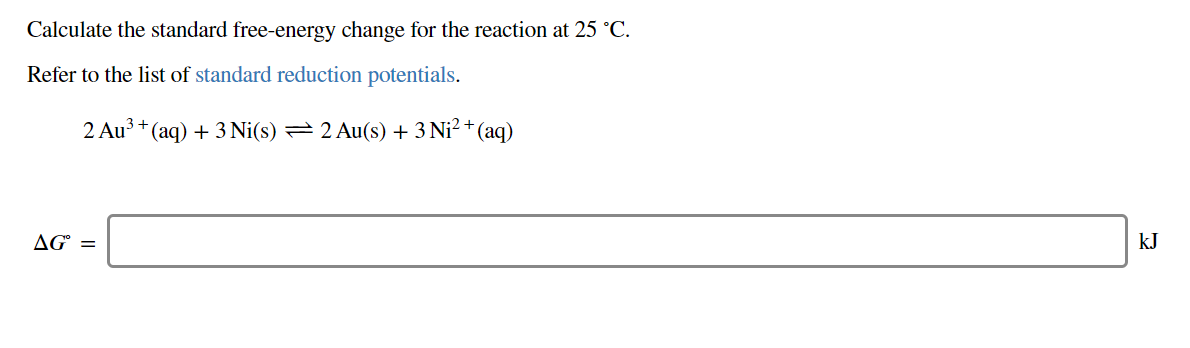# Calculate the standard free-energy change for the reaction at 25 °CRefer to the list of standard reduction potentials2 Au3 (aq)3Ni(s) 2 Au(s) 3Ni2+ (aq)AG=kJ

Question
46 viewshelp_outlineImage TranscriptioncloseCalculate the standard free-energy change for the reaction at 25 °C Refer to the list of standard reduction potentials 2 Au3 (aq)3Ni(s) 2 Au(s) 3 Ni2+ (aq) AG= kJ fullscreen
check_circle

Step 1

The standard electrode potential of a reaction is calculated by expression (1), in which E0cell is the standard electrode potential, E0C is the standard reduction potential at cathode, and E0A is the standard reduction potential at anode.

The relation between standard free energy change and cell potential of a reaction is given by equation (2), in which, ∆G0 is the standard free energy change, E0cell is the electrode potential of the cell, n is the number of electrons transferred, and F is faraday’s constant.

Step 2

The given reaction is,

2Au3+(aq) + 3Ni(s) → 2Au(s) + 3Ni2+(aq)

In the given reaction, nickel undergoes oxidation and Au undergoes reduction. Therefore, Ni is present at anode and Au is present at cathode.

At anode:       3Ni(s) → 3Ni2+(aq) + 6e-               E0 = -0.23 V

At cathode:    2Au3+(aq) + 6e- → 2Ni2+(aq)         E0 = +1.498 V

The electrode potential of the cell is calculated using equation (1),

Step 3

The number of electrons exchanged in the given reaction is 6 and 1 V = 1 J/C.

The sta...

### Want to see the full answer?

See Solution

#### Want to see this answer and more?

Solutions are written by subject experts who are available 24/7. Questions are typically answered within 1 hour.*

See Solution
*Response times may vary by subject and question.
Tagged in

### Chemistry